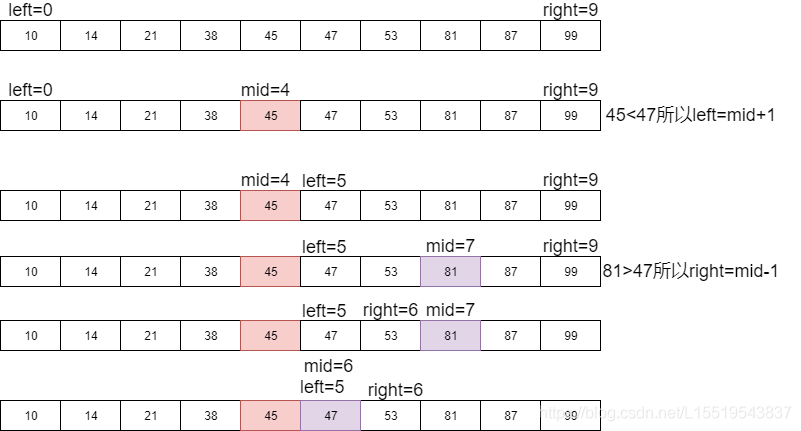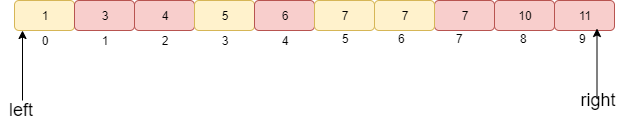# 手撕二分查找及其变种，就是干!# 一、初探二分查找

>在面试的时候，尤其的一面，感觉让你手写二分，还真的不一定就能很快写出来，所以在此总结分享给大家## 1 二分查找是什么？

”查找“顾名思义是在一堆数去找出我们需要的数，但是我们又想更快的找出我们需要找的数，所以我们就尽量的减少查找比较的次数。"二分"就是分成两份来减少我们查找次数。# 二 、二分的注意事项

1 二分查找要求数据必须是有序的。

2 二分查找依赖于数组随机查找的特性，要求内存连续

# 三 、二分的实现

1 第一种小白写法

int BinarySerach(vector<int>& nums, int target) {    int left = 0, right = nums.size();    while (left < right) {        int mid = (left+right)/2;        if (nums[mid] == target) return mid;        else if (nums[mid] < target) left = mid + 1;        else right = mid;    }    return -1;}2 方法二优化版>哪有这么简单的事儿，大多数的笔试面试中可能会出现下面的几种情况。

# 四 、二分的各种变种1 查找第一个值等于给定值的情况(查找元素 7)

>首先 7 与中间值 a比较，发现小于 7，于是在 5 到 9 中继续查找，中间 a=7,但是这个数 7 不是第一次出现的。那么我们检查这个值的前面是不是等于 7，如果等于 7，说明目前这个值不是第一次出现的 7，此时更新 rihgt=mid-1.ok 我们看看代码

int BinarySerach(vector<int>& nums, int n,int target) {    int left = 0, right = n-1;    while (left <= right) {        int mid = left+((right-left)>>1);        if (nums[mid]>value)        {        	right=mid-1;        } else if(nums[mid]<value)        {        	left=mid+1;        }else        {        	if((mid==0)||(nums[mid-1]!=value))        	{        		return mid;        	}else        	{        		left=mid-1;        	}        }    return -1;}

2 查找最后一个值等于给定值的情况

int BinarySerach(vector<int>& nums, int n,int target) {    int left = 0, right = n-1;    while (left <= right) {        int mid = left+((right-left)>>1);        if (nums[mid]>value)        {        	right=mid-1;        } else if(nums[mid]<value)        {        	left=mid+1;        }else        {        	if((mid==n-1)||(nums[mid+1]!=value))        	{        		return mid;        	}else        	{        		left=mid+1;        	}        }    return -1;}

3 查找第一个大于等于给定值的情况

1 如果 nums[mid]小于要查找的值，那么我们需要查找在[mid+1,right]之间，所以此时更新为 left=mid+1

2 如果 nums[mid]大于给定值 value,这个时候需要查看 nums[mid]是不是我们需要找的第一个值大于等于给定值元素，如果 nums[mid]前面没有元素或者前面一个元素小于查找的值，那么 nums[mid]就是我们需要查找的值。相反

3 如果 nums[mid-1]也是大于等于查找的值，那么说明查找的元素在[left,mid-1]之间，所以我们需要将 right 更新为 mid-1

int BinarySerach(vector<int>& nums, int n,int target) {    int left = 0, right = n-1;    while (left <= right) {        int mid = left+((right-left)>>1);        if (nums[mid]>=value)        {        	if(mid==0||nums[mid-1]<value)        	{        		return mid;        	}else        	{        		right=mid-1;        	}        }else        {        	left=mid+1;        }    return -1;}

4 查找最后一个小于等于给定值的情况

1 如果 nums[mid]小于查找的值，那么需要查找的值肯定在[mid+1,right]之间，所以我们需要更新 left=mid+1

2 如果 nums[mid]大于等于给定的 value，检查 nums[mid]是不是我们的第一个值大于等于给定值的元素

int BinarySerach(vector<int>& nums, int n,int target) {    int left = 0, right = n-1;    while (left <= right) {        int mid = left+((right-left)>>1);        if (nums[mid]>value)        {        	right=mid-1;        }else        {        	if(mid==n-1||(nums[mid+1]>value))        	{        		return mid;        	}else        	{        		left=mid+1;        	}        }    return -1;}

## 六 结尾## 评论